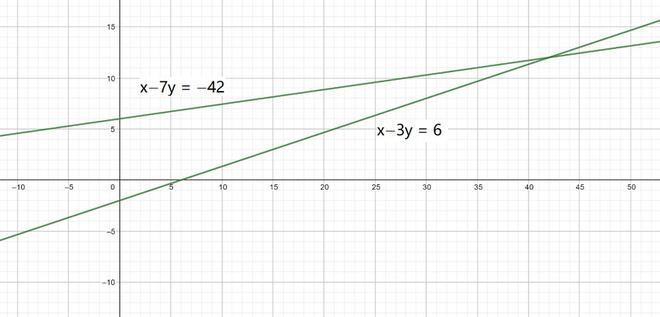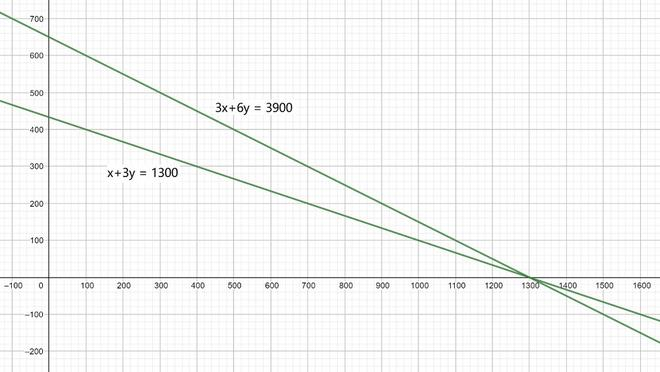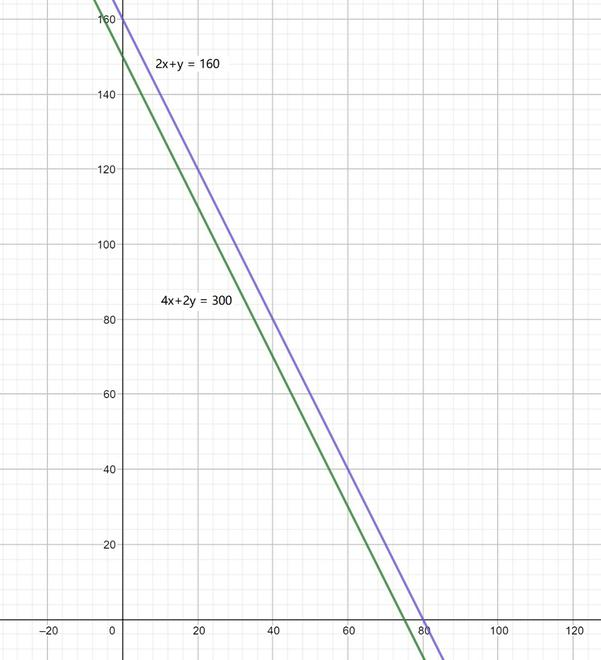Open in App
Not now

# Class 10 NCERT Solutions- Chapter 3 Pair of Linear Equations in Two Variables – Exercise 3.1

• Last Updated : 21 Feb, 2021

### Question 1. Aftab tells his daughter, “Seven years ago, I was seven times as old as you were then. Also, three years from now, I shall be three times as old as you will be.” (Isn’t this interesting?) Represent this situation algebraically and graphically.

Solution:

Present age of Aftab = x

And, the present age of his daughter = y

Seven years ago,

Age of Aftab = x-7

Age of his daughter = y-7

Three years after,

Age of Aftab = x+3

Age of his daughter = y+7

Here, According to the given condition,

x−7 = 7(y−7)

x−7 = 7y−49

x−7y = −42         ………………………(I)

To draw the line for Eqn. (I), we need at least two solutions of the equation, So, we can use the following table to draw the graph:

And, According to the another given condition,

x+3 = 3(y+3)

x+3 = 3y+9

x−3y = 6       …………..…………………(II)

To draw the line for Eqn. (II), we need at least two solutions of the equation, So, we can use the following table to draw the graph:

The graphical representation of Eqn. (I) and Eqn. (II) is:### Question 2. The coach of a cricket team buys 3 bats and 6 balls for ₹ 3900. Later, she buys another bat and 3 more balls of the same kind for ₹1300. Represent this situation algebraically and geometrically.

Solution:

The cost of a bat = ₹ x

And, the cost of a ball = ₹ y

Here, According to the Given condition,

3x+6y = 3900 …………………….(I)

x+3y = 1300 …………………….(II)

To draw the line for Eqn. (I), we need at least two solutions of the equation, So, we can use the following table to draw the graph:

To draw the line for Eqn. (II), we need at least two solutions of the equation, So, we can use the following table to draw the graph:

The graphical representation of Eqn. (I) and Eqn. (II) is:### Question 3. The cost of 2 kg of apples and 1kg of grapes on a day was found to be ₹ 160. After a month, the cost of 4 kg of apples and 2 kg of grapes is ₹300. Represent the situation algebraically and geometrically.

Solution:

The cost of 1 kg of apples = ₹ x

And, cost of 1 kg of grapes = ₹ y

Here, According to the given conditions,

2x+y = 160 ………………………(I)

4x+2y = 300 ………………………(II)

To draw the line for Eqn. (I), we need at least two solutions of the equation, So, we can use the following table to draw the graph:

To draw the line for Eqn. (II), we need at least two solutions of the equation, So, we can use the following table to draw the graph:

The graphical representation of Eqn. (I) and Eqn. (II) is:My Personal Notes arrow_drop_up